### Home > CALC > Chapter 10 > Lesson 10.4.2 > Problem10-154

10-154.
1. An object moves along the x­-axis with an initial position of x(0) = 2. The velocity of the object when t > 0 is given by the equation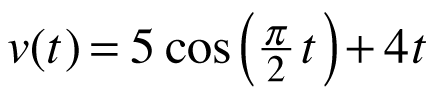. Homework Help ✎

1. What is the acceleration of the object when t = 4?

2. What is the total distance the object covers during the interval 0 ≤ t ≤ 4?

3. What is the position of the object when t = 4?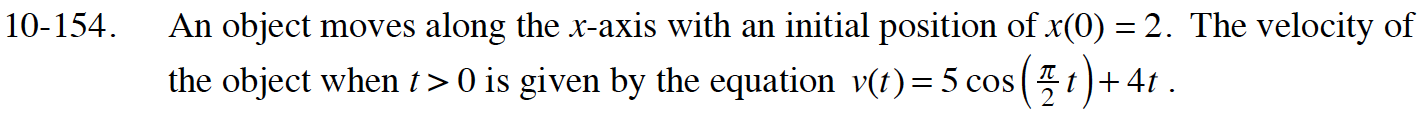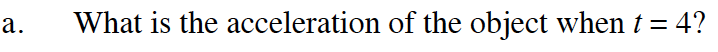This is v′(4).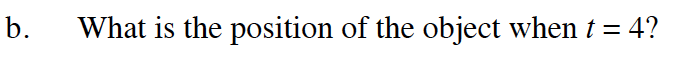This is x(4) where:

$x(t)=\int v(t)dt$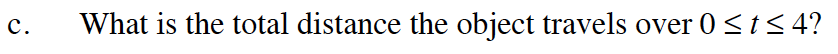This is x(4) – x(0).